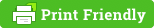# System converter onlinehttps://www.convert-me.comEnglish -Russian

Convert-me

Online unit converter.

In addition to the classic (basic) weight, mass, length ... on the site you can convert ancient units of measure, engineering, computer and others.https://convertworld.comEnglish -Russian

Convertworld

If we need to convert miles to kilometers, gallons to liters ... this site will be the most it.
The site is Swedish, there is Russian, the style is old, simple. But the main thing here is the base and fast work.
The base is beautiful, let's say we need to find out the rate of some small currency, and everywhere rubles, dollars and euros. And we need a shekel: click the Currency, shekel and a list of all currencies indicating how much the shekel is in rubles and Etc.
We enter our sum, the result changes without reloading in real time.

It seems to me that any ordinary user will find anything here for sure.

• Currency
• Pressure
• Translate
• Digits
• Angle
• Frequency
• Paper Size
• Dimensions
• TV and Monitors
• Length
• Volume
• Area
• Mass
• Strength
• Force moment
• Body mass index (BMI)
• Speed ​​
• Acceleration
• Wind speed
• Shoe size
• Clothing: dresses And suits
• Time zones
• Time
• Pregnancy calendar
• Density
• Molar mass
• Amount of substance
• Kinematic viscosity
• Dynamic viscosity
• Bandwidth
• Data storage
• Electric capacity
• Electric charge
• Electricity
• Electrostatic potential
• Electrical conductivity
• Electrical resistance
• Inductance
• Energy
• Fuel consumption
• Capacity
• Temperature
• Mass flow rate
• Volume flow
• Magnetic field
• Magnetic force
• Magnetic flux
• Magnetic force
• Illumination
• Brightness
• Light energy
• Luminous flux
• Light intensity
• Equivalent dose
•